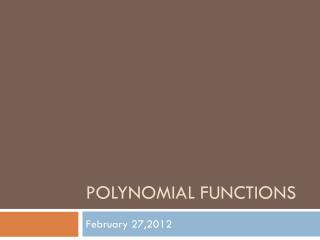DownloadDownload PresentationPolynomial Functions

# Polynomial Functions

Télécharger la présentation## Polynomial Functions

- - - - - - - - - - - - - - - - - - - - - - - - - - - E N D - - - - - - - - - - - - - - - - - - - - - - - - - - -
##### Presentation Transcript

1. Polynomial Functions February 27,2012

2. What is a polynomial function? • What is a polynomial? The sum or difference of two or more algebraic terms (monomials) • A polynomial equation used to represent a function is called a polynomial function Example: f(x) = 4x2 – 3x + 2 f(x) = 2x3 + 4x2 – 5x + 7 Quadratic Function Cubic Function

3. Do Now: What does it mean to evaluate a function? Plug in or substitute what is in the parenthesis for x in the function • Evaluate p(2) • p(x) = x2+ 3x + 4 p(2) = 22 + 3(2) + 4 = 14 • Evaluate p(b+1) • p(x) = x2 + 3x + 4 p(b+1) = (b+1)2 + 3(b+1) + 4 = b2 + 2b + 1 + 3b + 3 + 4 = b2 + 5b + 8

4. Vocabulary • Degree - refers to the highest exponent of a function • Leading Coefficient - is the coefficient of the term with the highest degree Example: What is the degree and leading coefficient of: 5x2 + 2x + 1 7x4 + 3x2 + x - 9 D: 2, LC: 5 D: 4, LC: 7

5. Graphs of polynomial functions • What relationship is there between the degree of the function and the maximum number of zeros? • The degree tells you how many roots you have • What do you notice about the degree and number of humps/ curves in the graph?The degree is one more than the number of humps

6. End Behavior • What happens to the graph as xapproaches ±∞? • This is represented by • x  + ∞ and x  - ∞ • x approaches positive infinity • X approaches negative infinity • End behavior expressed • f(x)  –∞, as x  –∞ • f(x)  + ∞, as x  +∞

7. For any polynomial function, the domain is all real numbers • For any polynomial function of odd degree, the range is all real numbers • The graph of any odd degree function has at least one x intercept

8. Put it all together • f(x)  - ∞, as x  - ∞ and f(x)  - ∞, as x  + ∞ • degree of 4 represents an even-degree polynomial function • 2 real zeros

9. ExitSlip

10. Agenda • Do Now – Determine end behavior through graph • Review HW • Notes – Determining end behavior through equation • Class work

11. Do Now a) f(x) +∞ , as x +∞ f(x) +∞ , as x -∞ b) Even c) No real zeros

12. End Behavior: UpUp END BEHAVIOR Degree: Even Leading Coefficient: +

13. End Behavior: DownDown Leading Coefficient: END BEHAVIOR Degree: Even

14. End Behavior: Down Up END BEHAVIOR Degree: Odd Leading Coefficient: +

15. End Behavior: UpDown Leading Coefficient: END BEHAVIOR Degree: Odd

16. End Behavior

17. END BEHAVIOR PRACTICE Give the End Behavior:

18. Down Down Up Up Down Up Up Down END BEHAVIOR PRACTICE Give the End Behavior:

19. Do Now • What will the end behavior of the following polynomial functions be? (hint: find the degree and leading coefficient) • y = – x2 + 6x – 5 • y = x5 – 3x2 + 2x – 6

20. Agenda • Do Now- Review • Notes • Behavior on intervals • Group Work Activity • Explain Project • Homework

21. Today’s Objective: • Identify increasing, decreasing, and constant intervals

22. Increasing, Decreasing, and Constant Intervals • An interval is the set of all real numbers between two given numbers • A function f is increasing on an interval if as xincreases, then f(x) increases. • A function f is decreasing on an interval if as xincreases, then f(x) decreases. • A function f is constant on an interval if as xincreases, then f(x) remains the same.

23. Increasing, Decreasing, Constant Intervals Find the interval(s) over which the interval is increasing, decreasing and constant?

24. f(x) is increasing in the intervals Increasing, Decreasing, Constant Intervals f(x) is decreasing in the interval (-1,1).

25. Today’s Agenda • Do Now • Review Home work • Intervals • Review Notes • What do they say about… • Class work • End behavior worksheet

26. Do now Find the interval(s) over which the interval is increasing, decreasing and constant?

27. f(x) is decreasing over the intervals f(x) is increasing over the interval (3,5). f(x) is constant over the interval (-1,3).